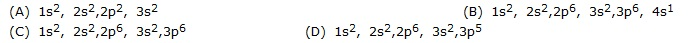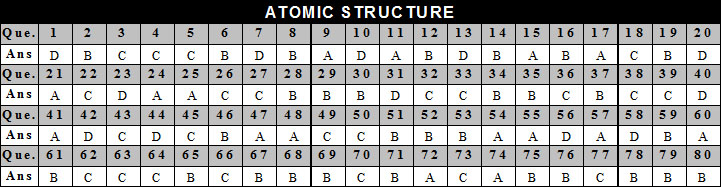Welcome to Study Material Solution
Leave Comment

### NCERT 9TH CLASS SCIENCE CHAPTER STRUCTURE OF ATOM

INTRODUCTION

According to John Dalton : All matter was composed of small  particle called atom.
Atom is a Greek word and its meaning Indivisible i.e. an ultimate particles which cannot be further subdivided.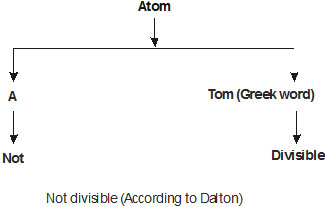According to Dalton's theory -
(1)   Atom is the smallest indivisible part of matter which takes part in chemical reaction.
(2)  Atom is neither be created nor be destroyed.
(3)  Atoms of the same element are similar in size, mass and characteristics ; however, atoms of different elements have different size, mass and characteristics.
l    An atom is made up of three subatomic particle electron, proton & neutrons. These three particles are called fundamental particles of matter.

##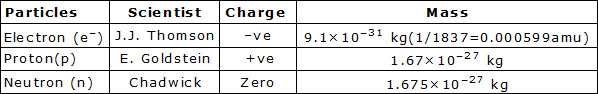DO YOU KNOW :  Mass of Proton is  2000 times more than electron        ATOMIC STRUCTURE

There are two parts of atom :
(i) Nucleus                     (ii) Outer part

Nucleus : The size of nucleus of atom is very small in which neutrons and protons are present so the almost entire mass of the atom is situated in nucleus.

Protons & neutrons present in the nucleus are collectively termed as nucleus. Number of neutrons is called mass number element. Size of the nucleus
in the atom is 10–15m.
Mass number (A) = Number of protons in the nucleus (p) + Number of Neutrons (n).
Outer Part : In the outerpart, electrons move around the nucleus in fixed orbits. These orbits are called energy levels.

### CATHODE RAYS

(Discovery of e–) :
In 1859 "Julius Plucker" started the study of conduction of electricity through gases at low pressure in a discharge tube

. When a high voltage of 10,000 volts or more was impressed across the electrodes, some sort of inversible rays moved from

the negative electrodes to the positive electrode. Since the negative electrode is referred to as cathode. These rays were called cathode rays.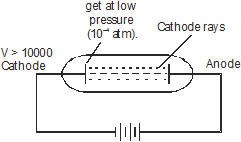### Properties of cathode rays :

• These rays travels in straight line away from cathode with very high velocity ranging from 107 to 109 m/sec.
•  A shadow of metallic object placed in the path is cast on the wall opposite to cathode.
•  Cathode rays cause green fluorescence on glass surface, i.e the glasss surface on which the cathode rays strike show a coloured shine.
•  Cathode rays consist of matter particles, and posses energy by the virtue of their mass and velocity.
•  Cathode rays set a paddle wheel into motion when it is placed in the path of these rays. This is due to the
•  impact of the particles of the cathode rays on the blades of the paddle wheel.

###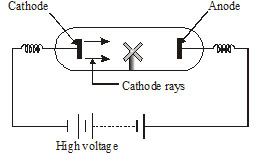Cathode ray particles strike the blades of the paddle wheel, and set it into motion

• These rays are deflected by the electric of magnetic fields when the rays are passed between two electrically charged plates,
• these are deflected towards the positively charged plates. It shows that   cathode rays carry negative charge.
•  These particles carrying negative charge. These particles carrying negative charge were called negatrons by thomson. The name negatron was
changed "Electron by   Strong".
•  These rays produce heat energy when they collide with the matter. It shows that cathode rays possess kinetic energy which is converted into
heat energy when stopped by matter.
•  These rays affect the photographic plate.
•  Cathode rays can penetrate the thin foil of solid materials.
•  Cathode rays can ionize the gases through which they pass.
•  The nature of cathode rays independent of

(a) The nature of cathode
(b) The gas in discharge tube
Ques. What are cathode rays ?                                                                [NCERT]

### Measurement of e/m for electron :

In 1897 J.J. Thomson determine the e/m value, charge/mass of the electron by studying the deflection of cathode rays in electric & magnetic fields.
The value of e/m has been found to be –1.7588 × 108 coulomb/unit. The absolute value of the charge in an e– was measured by R.A.
Milikan 1909 by the Milikan's oil drops experiment.

### ANODE RAYS OR CANAL RAYSIt has been established that electron is a negatively charged particle present in all the atoms. As an atom is electrically neutral,

there must be some positively charged particle present in the atom to neutralize the negative charge of the electrons.

It has been confirmed by experiment "Goldstein" in 1886 discovered the existence of  a new type of rays in discharge tube

. He carrried out experiment in discharge tube containing perforated cathode. These rays moved towards cathode and passed through
the perforation in the cathode.

•  Initially these ray were called canal rays because they pass through the canals or holes of the cathode.
•  These rays are also called anode rays since they originate from the anode side.
•  Anode rays are positively charged, therefore these were named positive rays by Sir J.J.  Thomson.

### Characteristics of anode rays :

Anode rays travel in straight lines. These rays rotate the light paddle wheel placed in their path

. Anode rays are deflected by magnetic or electric field towards negatively charged plate. This indicates that these rays are positively charged.

• The anode rays affect photographic plate.
•  The nature of anode rays depends upon the type of gas used. The charge (e) to mass (m) ratio (e/m) of anode rays particle is different for
different gases. The value of e/m is maximum for hydrogen gas.
•  The positive rays obtained from hydrogen are made up the same type of positive particles. These particles are known as protons.

In the discharge tube the atoms of gas lose negatively charged electrons. These atoms, thus acquire a positive charge . The positively
charge particle produced from hydrogen gas were called "protons".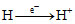### (Proton)    Characteristics of proton :

A proton is a fundamental particle of atom carrying one unit positive charge of having mass nearly equal to the mass of an atom of hydrogen.

The protons are present in the nucleus of the atom.
Charge of proton :  Proton is a positively charged particle. The charge on a proton is equal but opposite to that on an electron.

Thus the magnitude of charge on a proton is + 1.602 × 10–19 coulomb/unit.  The mass of proton is equal to the mass of hydrogen atom.

Ques. If an atom contains one electron and one proton, will it carry any charge or not ? [NCERT]

### THOMSON MODEL OF ATOM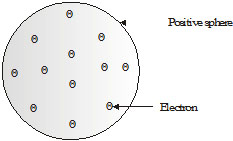Fig. Thomson's model of the Atom

l   Detailed model of the atom was first of all proposed by Sir J.J. Thomson.
l   Thomson proposed that an atom consist of a uniform sphere of positive charge in which the electrons are distributed more or less uniformly.
l   Thomson proposed the model of an atom to be similar to that of a Christmas pudding. The electrons,

in a sphere of positive charge, were like currants (dry fruits) in a spherical Christmas pudding.

We can also think of a watermelon, the positive charge in the atom is spread all over like the red edible part of the watermelon,

while the electron are sudden in the positively charged sphere, like the seeds in the watermelon.

Ques. On the basis of Thomson's model of an atom, explain how the atom is neutral as a whole.[NCERT]

### Drawback of the Thomson Model :

l   An important drawback of this model is that the mass of the atoms is considered to be evenly spread over that atom.
l   It is static model. It does not explain the movement of electron.
l   It could not explain the stability of an atom.

### RUTHERFORD'S  MODEL OF THE ATOM (DISCOVERY OF NUCLUES)

In 1911, scientist "Ernest Rutherford" gave a new picture for the structure of atom by his a-particle scattering experiment & proposed

the structure of atom. a particle are charged particles having 2 unit of positive charge and 4 units of mass, that is a-particle (2He4) are
doubly charged helium atom (He+2)

### Rutherford Experiment :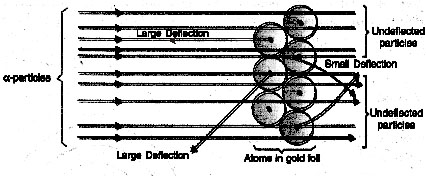• When fast moving alpha particles  strike very thin gold foil in vaccum, it is found that :
• Most of the fast moving a-particles passed straight through the gold foil, without any deflection from their original part.
• Some of the a-particles were deflected by the foil by small angles and few are deflected through large angles.
•  A very few alpha particles completely rebound on hitting the gold foil and turn back on their path.

### Conclusion of Rutherford experiment :

Rutherford concluded from the a-particle scattering experiment that

•  Most of the space inside the atom is empty because most of the a-particle passed through the gold foil without getting deflected.
•  Very few particles were deflected from their path, indicating that the positive charge of the atom occupies very little space.
•  A very small fraction of a-particles were deflected by 180°, indicating that all the positive charge and mass of the gold atom were
concentrated in a very small volume within the atom.
•  On the basis of this experiment, Rutherford put forward the nuclear model of an atom, which has the following features.
•  There is a positively charged centre in an atom called the nucleus. Nearly all the mass of the an atom resides in the nucleus.
•  The electrons revolve around the nucleus in well-defined orbit.
•   The size of the nucleus is very small as compared to the size of the atom.

Ques. On the basis of Rutherford's model of an atom, which subatomic particle is present in the nucleus of an atom ?                                        [NCERT]

DO YOU KNOW :  Radius of nucleus is 10–15m radius of atom is 10–10m.

### Drawback of the Rutherford Experiment :

According to Maxwell, if an electrically charged particle revolves around circular path, then it always radiate out energy Thus.

If an electron moves around the nucleus, it must continuously radiate out energy and hence, gradually more towards nucleus in a
spiral path, till it collide with nucleus.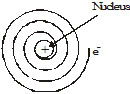### BOHR MODEL OF ATOM

Rutherford's Model of the atom was unable to explain certain observations with regard to the atom that is stability of the atom and the
occurence of the atomic spectra.
Neil's Bohr accepted Rutherford's idea that the positive charge and most of the mass of atom is concentrated in its nucleus with the
electrons present at some distance away.

It is a quantum mechanical model. This model was based on quantum theory of radiation or plank theory and classical law of physics.

### According to Bohr's Theory :

• Electrons revolve around the nucleus in well defined orbits or shells each shells having a definite amount of energy associated with the electrons in it.
Therefore these shells are also called energy levels.
• The energy associated with the electrons in an orbit increase as the radius of the orbit increase. These shell also known as K, L, M, N..............starting
from the one closest to the nucleus.
•  An electron in a shell can move to a higher or lower energy shell by absorbing or releasing a fixed amount of energy.
•   The amount of energy absorbed or emitted is given by differences energies associated with the two levels. Thus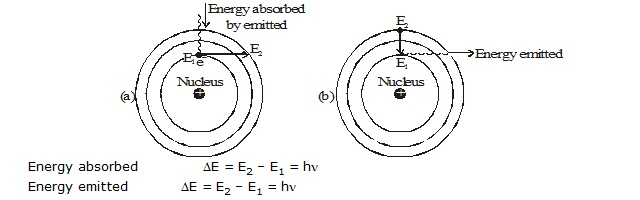where h is plank's constant and n is the frequency of the radiation.

###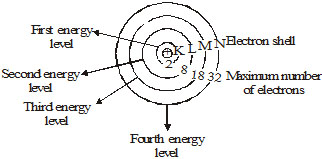Ques. Draw a sketch of Bohr's model of an atom with three shells.                         [NCERT]          DISCOVERY OF NEUTRON

In 1932, "Chadwick " bombarded berylium with a stream of a-particle. He observed that penerating radiations were produced

which were not affected by electric & magnetic field. These radiations consists of neutral particles, which were called neutron.
The nuclear reaction can be shown as as: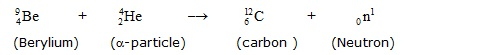In this equation the subscript stand for number of protons and the superscript for mass number. It is now clear that the neutron is
fundamental consitituent of atom and is located in the nucleus.
Characteristics of a neutron :
Mass : The relative mass of a neutron is almost equal to that of proton. In fact relative mass of neutron is 1.0087 (1.008) amu and that of
proton is 1.0073 (1.008) amu.
Charge : Neutron is electrically neutral and has no electric charge. With the discovery of neutrons, we can explain why the atomic mass of
helium is 4 amu. Atomic mass = Mass of proton + Mass of neutron.
Valency : An atom of each element has definite combining capacity of the element is called valency. The number of electron (e–) gained, lost
or shared by the atom of an element to complete octet stable :
Ex :- Ne20.
Valence electron :  The electron present in the outer most shell are called valence electron.
Ex. Na+ and N–

Ques. How will you find the valency of chlorine, sulphur and magnesium ?                 [NCERT]

### ATOMIC NUMBER AND MASS NUMBER

Atomic number (Z)  :

It is denoted by 'Z'. Thus atomic number = No. of protons = No. of electrons for hydrogen Z = 1 because in hydrogen atom only one
proton is present in the nucleus.
Therefore, the atomic number is defined as the total number of protons  present in the nucleus of an atom.
Ex. 6C12 means atomic number of carbon is 6. Nucleus of carbon has 6 protons. Nucleus of carbon has 6 unit positive charge. There are 6
electrons, revolving round the nucleus of carbon.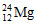The mass number of magnesium is 12. The total number of protons and neutrons in the nucleus of magnesium is 24 number of protons is 12.
Number of neutrons is = 24 – 12 = 12.
Mass Number (A) : The mass number of an atom is defined as the sum of the total number of protons and

neutrons present in the nucleus of an atom. For example, mass of carbon is 12u because it has 6 proton and 6 neutron that is 6u. + 6u. = 12u.
Mass number : Number of protons + number of neutron for eg.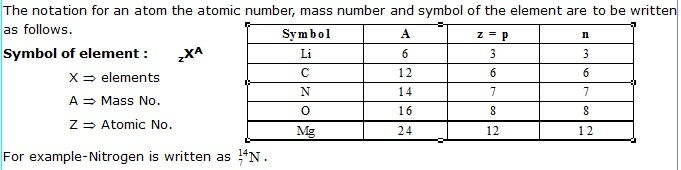For example-Nitrogen is written as .
Ques. If number of electrons in an atom is 8 and number of protons is also 8, then  (i) what is the atomic number of atom (ii) what is the
charge of the atom ?                                 [NCERT]
Ques. Find out the mass number of oxygen and sulphur atom.                                 [NCERT]

ISOTOPES
Isotopes are atoms of the same element, having the same atomic number but different mass number.
For example isotopes of hydrogen atom, namely protium (1H1 ) deuterium(1H2) tritium (1H3).
The atomic number of each one is 1, but the mass number is 1, 2, and 3 respectively.
Exampe of Isotopes :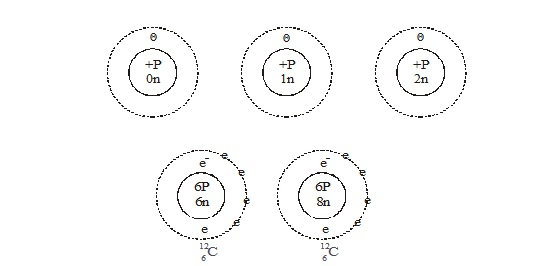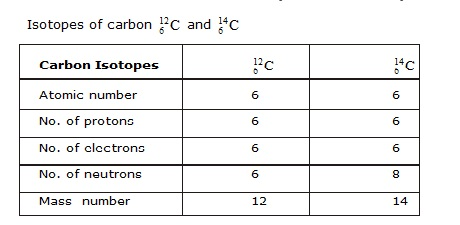Applications of Isotopes :
Since the chemical properties of all isotopes of an element are the same but same isotopes have special properties which find
them usefulin various fields. Some of them are :

•  Uranium () is used as a fuel in nuclear reactors.
•  Cobalt () is used in the treatment of cancer.
•  odine () is used in the treatment of goitre.
•  Sodium () is used for differentiating cancerous tissues from the normal tissues.
•  Carbon  is used in dating of fossil samples.

How to calculate the atomic mass of an element from the mass number of its isotopes.
The atomic mass of an element is the weighted arithmetic mean of the atomic masses of its isotopes present in the sample of the
element.Let us consider a sample of an element X containing its two isotopes X1 and X2.
Atomic mass of the element X =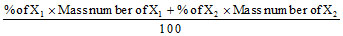Ex. This method is illustrated by taking the case of chlorine. The two isotopes of chlorine, and  occur in the ratio 3 : 1. Then,
Atomic mass of chlorine :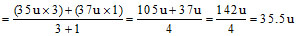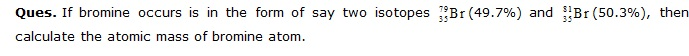ISOBARS
Atoms of different elements with different atomic number which have the same mass number, are known as isobars.
For example : Calcium and argon
20Ca40          18Ar40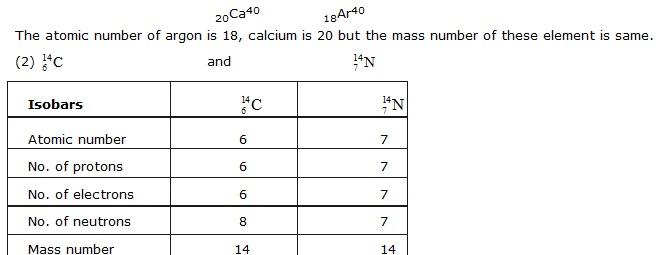Ques. Write the electronic configuration of any one pair of isotopes and isobars.         [NCERT]
Order of filling of electrons in orbitals :
There are different rules for filling electrons in subshells. They are described as follows.
l    Pauli's exclusion principle.
l    Aufbau principle
l    (n + l) rule
l    Hund's maximum multiplycity rule.
Pauli's Exclusion Principle :
l    According to Pauli's exclusion principle an orbital cannot accomodate more than two electrons.

These two electrons should have opposite spins. eg. :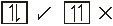Aufbau Principle :
Aufbau is a German word and its meaning building up. Aufbau principle gives a sequence in which various subshell are filled up depending on

the relative order of the energies of various subshell. The subshell with minimum energy is filled up first, when this subshell obtained maximum no

. of e– or capacity of electrons then the next   subshell of higher energy starts fillings.
The order of filling of different sub-shell in represented diagramatically as follows :
Simmon's Array :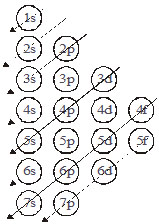Hund's Maximum Multiplicity Rule :
According to hund's rule electrons are distributed among the orbitals of subshell in such a way to give maximum number of
unpaired electronwith parallel spin.i.e.

in a subshell pairing of electons will not start until and unless all the orbitals of that subshell will get one electron each with same spin.
For example :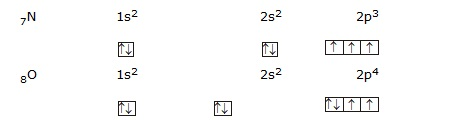(n + l) Rule : According to it the sequence in which various subshells are filled up can also be determined with the help
of (n + l) value for a given subshell.

The subshell with lowest (n + l) value is filled up first when two or more subshells have same (n + l) value then the subshell with lowest
value of n is filled up first.

Sub Shell        n        l        n +l
1s            1        0        1
2s            2        0        2
2p            2        1        3    (1)
3s            3        0       3]  (2)
3p            3        1       4    (1)
4s            4        0       4]   (2)
3d           3        2       5    (1)
4p           4        1        5    (2)
5s           5        0       5]  (3)
4d           4        2       6    (1)
5p            5       1       6    (2)
6s            6        0      6]   (3)

### ELECTRON DISTRIBUTION IN DIFFERENT ORBIT (SHELL)

The  distribution of electron into different orbits of an atom was suggested by Bohr and Bury. The following rules are followed for writing

the number of electrons in different energy level or shell. The maximum number of electrons present in a shell is given by the formula 2n2. Where

'n' is the orbit number or energy level index 1,2,3. Hence the maximum number of electrons in different shells are as follows.
First orbit  (K-shell)
2 × 12 = 2
Second orbit  (L-shell) = 2 × 22 = 8
Third orbit  (M-shell) = 2 × 32 = 18
Fourth orbit  (N-shell) = 2 × 42 = 32
The maximum number of electrons that can be accommodated in the outermost orbit is 8.
l    Electron are not accommodated in a given shell. unless the inner shell are filled. That is, the shell are filled in a step-wise manner.
Orbitals : The three dimensional area around the nucleus of an atom where the probability of finding the moving electrons is
maximum is called orbital.

### ROUND UP

•    Discharge tube studies by J.J. Thomson and other led to the discovery of electron.
•    J.J. Thomson determined the value of charge/ mass ratio, e/m of the electron.
•    R.A. Millikan determined the value of charge on the electron.
•    Positive rays were discovered by E. Goldstein and these are also called canal rays.
•    Rutherford and his co-workers performed a-particle scattering experiments which led to the discovery of atomic nucleus.
•    Atoms of the same element which have the same atomic number but different mass number are called isotopes.
•    Atoms of different elements having same mass number but different atomic number are called isobars.
•    Bohr’s model of an atom gave the concept of orbits or shells. These are designeted as K, L, M, N,
•    Each orbit has a definite energy and that is why these are also called energy levels or energy shells.
•    Neutron was discovered by Chadwick.
•    The number of protons in an atom of an element is known as atomic number.
•    The sum of the number of protons and neutrons in an atom of the element is called mass number.
•    In general, the maximum number of electrons that can be present in any shell is 2n2 where n is the number of energy shell.
•    The distribution of electrons in different energy shells is called electronic configuration. Electronic configuration of atoms are written
with the help of K, L, M, etc.
•    The electrons present in the outermost orbit (or valence shell) are called valence electrons.
•    The valency of atom is either no. of electrons in valence shell or 8 – no. of electrons in the valence shell.

### NCERT QUESTIONS WITH SOLUTIONS

Q.1    What are canal rays?
Ans.    The anode rays are also called canal rays because anode rays were passed through perforated cathode/hole which were called canal by
Goldstein. Therefore, he named these rays as canal rays.

Q.2    If an atom contains one electron and one proton, will it carry any charge or not?
Ans.    No, the atom will be neutral as the negative charge of one electron is cancelled out by the positive charge of one proton. So,
the resultant charge will be zero.

Q.3    On the basis of Thomson’s model of an atom, explain how the atom is neutral as a whole.
Ans.    According to Thomson’s atomic model, the embeded electrons are equal to the positive charge on sphere, so net charge on the atom is zero.

Q.4    On the basis of Rutherford’s model of an atom, which sub-atomic particle is present in the nucleus of an atom?
Ans.    Protons are the sub-atomic particles which are present in the nucleus on the basis of Rutherford’s model of an atom.

Q.5    Draw a sketch of Bohr’s atomic model with three shells.
Ans.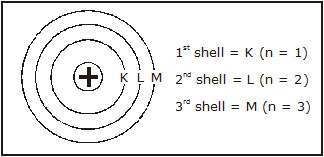Q.6    What do you think would be the observation if the a-particle scattering experiment is carried out using a foil of metal other than gold?
Ans.    If a-particles scattering experiment is carried out with other metals, i.e., heavy metals like platinum or silver then experiment may be almost
same as that with gold. But if lighter metals are taken then the a-particles may knock out the nulceus and they may not be returned back or deflected.

Q.7    Name the three sub-atomic particles of an atom.
Ans.    The sub-atomic particles are electrons, protons and neutrons.

Q.8    Helium atom has an atomic mass of 4 u and two protons in its nulceus. How many neutrons does it have?
Ans.    Atomic mass = 4, Number of protons = 2 = Atomic number
So, number of neutrons = Mass number – Atomic number = 4 – 2 = 2

Q.9    Write the distribution of electrons in carbon and sodium atoms.
Ans. For carbon atom: Atomic number = 6
Thus total number of electrons = 6
Hence, K = 2, L = 4.
For sodium atom: Atomic number = 11
Total number of electrons = 11
Thus K = 3, L = 8, M = 1

Q.10    If K and L shells of an atom are full, then what would be the total number of electrons in the atom?
Ans.  Maximum number of electrons in K shell = 2  × 12 = 2
Maximum number of electrons in L shell = 2 × 22 = 8
Hence, total number of electrons = 2 + 8 = 10

Q.11    How will you find the valency of chlorine, sulphur and magnesium?
Ans.    Atomic number of chlorine = 17Q.12    If number of electrons in an atom is 8 and number of protons is also 8, then:
(a) What is the atomic number of the atom? and
(b) What is the charge on the atom?
Ans.    (a) Atomic number = Number of protons = 8
(b) The given atom has equal number of proton and electrons. So, net charge will be zero and the atom will be neutral.

Q.13    For the symbol H, D and T, tabulate three sub-atomic particles found in each of them.
Ans.    The three isotopes of hydrogen which can be represented asH (Proteium),        Atomic number = 1
Mass number = 1
Number of neutrons = A – Z = 1 – 1 = 0
D (Deutrium),        Atomic number = 1
Mass number = 2
Number of neutrons = A – Z = 2 – 1 = 1
T (Tritium),        Atomic number = 1
Mass number = 3
Number of neutrons = A – Z = 3 – 1 = 2

Q.14    What are the limitations of Rutherford’s model of the atom?
Ans.    Limitations of Rutherford’s atomic model are:
He could not explain the stability of the atom, as he described that electrons revolve around the nucleus under the influence of positive charge of the nucleus.

But according to electron dynamics when a charged particle revolves around the opposite charge, its speed accelerates and it loses the energy and collapses.

Q.15  What are the limitations of J.J. Thomson’s model of the atom?
Ans.  The limitations of Thomson’s atomic model are:
(a) He could not explain the results of Rutherford’s alpha particle experiment.
(b) He could not explain the spectral properties of the atom.

Q.16    Write the electronic configuration of any one pair of isotopes and isobars.
.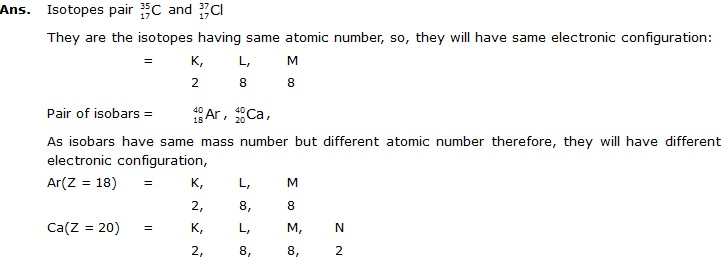Q.17    Compare the properties of electrons, protons and neutrons.
Ans.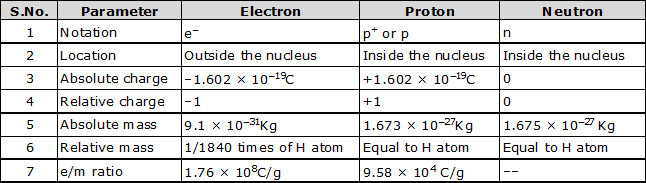Q.18    Describe Bohr’s model of the atom.
Ans.    Neils Bohr proposed his model in 1913 in order to overcome the shortcomings of Rutherford’s model. He gave the concept of orbits,
discrete states or energy levels.

Q.19    Compare all the proposed models of an atom given in this chapter.
Ans.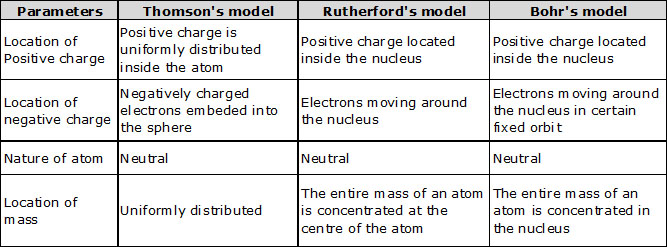Q.20    Define valency by taking examples of silicon and oxygen.
Ans.    The combining capacity of an element is called its valency or the number of electrons lost, gained or shared by an atom during bond
formation or chemical combination is called its valency.
For example, silicon (Z = 14)
Electronic configuration,    K,    L,    M
2,    8,    4
So, the valency = 4, as the last shell (valency shell) has 4 electrons.
Oxygen (Z = 8)
Electronic configuration–    K,    L
2,    6
The last shell or valence shell has 6 electrons, it will take 2 electrons to complete its octet, therefore the valency is 2.

Q.21    Explain with examples
(i) Atomic number                (ii) mass number
(iii) Isotopes                       (iv) Isobars
Give any two uses of isotopes.
Ans.    (i)    Atomic number(Z): The number of protons present in the nucleus of an atom represents its atomic number.
For example,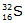Sulphur has 16 protons in its nucleus, its atomic number is 16.
(ii)    Mass number: Mass number(A) is the sum of number of protons and number of neutrons.
A = Number of protons + Number of neutrons
A = Z + n
For example, In aluminium, the  nucleus has 13 protons and 14 neutrons,
therefore, the mass number = 13 + 14 = 27
(iii)    Isotopes: They are the atoms of same elements which have same atomic number but different mass number. The difference
in mass number is due to the difference in number of neutrons.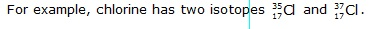.
(iv)    Isobars: They are the atoms of different elements which have same mass number but different atomic number, therefore, they
have different physical and chemical properties.
For example,  and
Uses of Isotopes:
(i)    Iodine –131 is used in treatment of thyroid cancer.
(ii)    C – 14 is used in estimation of age of fossils.

Q.22    Na+ has completely filled K and L shells. Explain.
Ans.    Atomic number of sodium = 11
Electronic configuration =    K,    L,    M
2    8    2
Sodium atom loses one electron to form Na+ ion.
As we know that K is the first shell and maximum electrons in 1st shell = 2 × 12 = 2
L is 2nd shell and the maximum electrons in 2nd shell = 2 × 22 = 8 electrons
Electronic configuration of Na+ is 2, 8. So, we find out the K and L shell of Na+ is completely filled.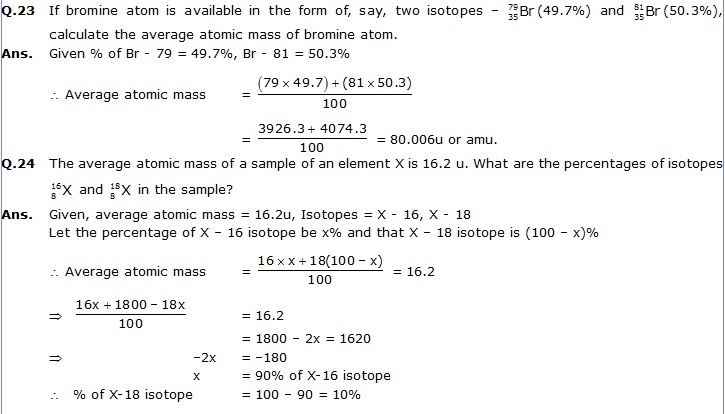Q.25    If X = 3, what would be the valency of the element? Also, name the element.
Ans.    Given Z = 3, the element is Li (lithium).
Now electronic configuration =    K,    L
2,    1
The last shell or the valence shell has 1 electron.
The valency = 1+

Q.26    Composition of the nuclei of two atomic species X and Y are given below:
=    X    Y
Protons    =    6    6
Neutrons    =    6    8
Give the mass numbers of X and Y. What is the relation between the two species?
Ans. According to the given composition:
Mass number = Number of protons + Number of neutrons
Mass number of X = 6 + 6 = 12
Mass number of Y = 6 + 8 = 14
These are isotopes of each other because they have same atomic numbers but different mass number.

Q.27    For the following statements, write (T) for true and (F) for false.
(a)  J.J. Thomson proposed that the nucleus of an atom contains only nucleons.
(b)  A neutron is formed by an electron and a proton combining together. Therefore, it is neutral.
(c)  The mass of an electron is about 1/1840 times that of proton.
(d)  An isotope of iodine is used for making of tincture iodine which is used as a medicine.
Ans.    (a)    F, as it was not stated by Thomson.
(b)    F, as neutrons are separate particles.
(c)    T, as this was proved experimentally.
(d)    F, as the iodine (I-131) is radioactive and costly, so, only simple iodine (I-127) and alcohol is used in tincture of iodine.

### EXERCISE I

Q.1    The first use of quantum theory to explain the structure of atom was made by –
(A) Heisenberg        (B) Bohr         (C) Plank    (D) Einstein

Q.2    Plum-Pudding model is known as–
(A) Rutherford Model
(B) Thomson's model
(C) Bohr Model
(D) All the above

Q.3    Nucleous consist of–
(A) Proton and electron
(B) Proton and neutron
(C) Neutron and electron
(D) Only neutron

Q.4    The number of valence electron in Na is –
(A) 1               (B) 2        (C) 3     (D) 4

Q.5    Mass number of element is –
(A) Number of protons in its nucleus                              (B) Number of electrons and protons in the atom
(C) Number of neutrons and protons in the nucleus        (D) Number of neutrons in the nucleus

Q.6    According to the Dalton's atomic theory –
(A) Electrons, proton and neutron are found in atom
(B) Atom is indivisible
(C) Atom of various element have same properties
(D) Atoms are destroyed in the chemical reaction

Q.7    Neutron is a fundamental particle which have–
(A) +1 unit charge and 1 unit mass         (B) No charge and 1 unit mass
(C) Have no charge and mass                (D) Have –1 unit charge and 1 unit mass

Q.8    No. of unpaired electron in 1s2.2s2.2p4
(A) 4                     (B) 2        (C) 0                  (D) 1

Q.9    The electron should be filled in the orbital in accordance with the increasing order of their energy. This statement is related with –
(A) AUfbau principle    (B) Pauli principle     (C) Hund's principle     (D) Plank's rules

Q.10    Which species does not contain neutron –
(A) H                     (B) Li+2          (C) C               (D) o

Q.11    Which of the following electronic configuration does obey aufbau principle –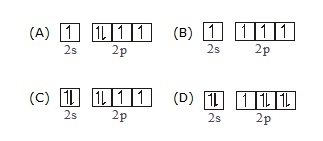Q.12    Atomic number of any element–
(A) Atomic weight divided by two
(B) Number of neutron in nucleus
(C) Nucleus weight
(D) Nuclear charge

Q.13    Lighest particle is –
(A) Neutron    (B) Electron       (C) Proton    (D) None of these

Q.14    Fundamental particle of atomic nucleus is–

(A) p, e–    (B) p, n, e         (C) p, n    (D) n, e

Q.15    The atomic size is nearly –
(A) 10–10cm    (B) 10–6m          (C) 10–7m    (D) 10–10m

Q.16    An atom which has a mass number of 14 or 8 neutrons is–
(A) Isotope of oxygen
(B) Isotope of oxygen
(C) Isotope of carbon
(D) Isobar of carbon

Q.17    Electronic configuration of p in ground state is–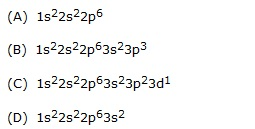Q.18    When a gas at reduced pressure is subjected to an electric discharge, the rays orignating from the negative electrode are–
(A) Anode rays     (B) Cathode rays      (C) x-rays    (D) Radiation ray,

Q.19    The maximum number of electrons that can be accomodate in the nth level is–
(A) n2                (B) 2n2                  (C) 4n2           (D) n

Q.20    Isotopes of an element are  the atom which have –
(A) The same number of neutrons in their nuclei            (B) The same mass number
(C) Different number of electrons in their orbit             (D) The same number of proton in their nuclei

Q.21    Number of valence electron in Ar are–
(A) 8                  (B) 18       (C) 19              (D) 20

Q.22    Rutherford's alpha-particle Scattering experiment was responsible for the discovery of–
(A) Proton    (B) Electron            (C) Neutron     (D) Atomic nucleus

Q.23    Isotopes of an elements have –

(A) different chemical properties
(B) different atomic
(C) The same physical properties
(D) Different number of neutrons

Q.24    Which one of the following is a correct electronic configuration of calcium–
(A) 2, 8, 8, 1    (B) 2, 6, 8, 2    (C) 2, 8, 8, 2    (D) 2, 8, 2, 8

Q.25    Which one of the following is a correct electronic configuration of chromium–
(A) 2, 8, 8, 6    (B) 2, 8, 8, 2, 4    (C) 2, 8, 8, 1,5    (D) 2, 8, 8, 2, 5

Q.26    and  differ from other in number of
(A) Electron    (B) Proton         (C) Neutron    (D) A & C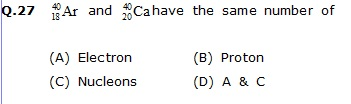Q.28    The total number of neutrons in zinc
(z = 30) with mass number 70 is -
(A) 40        (B) 20            (C) 36    (D) 38

Q.29    The sum of number of neutron and protons in one of the isotopes of hydrogen is-

(A) 3          (B) 2            (C) 5               (D) 6

Q.30    Deflection back of a few particles on hitting thin foil of gold shows that-
(A) Nucleus in heavy
(B) Nucleus in small
(C) Both (1) and (2)
(D) Electron create hinderance in the movement of a-particles.

Q.31    The number of electrons in the M-shell of the element with atomic number 24 is -
(A) 24              (B) 12            (C) 13            (D) 8

Q.32    Electronic configuration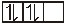violated
(A) Hund's rule     (B) Pauli's rule         (C) Aufbau rule      (D) All of the above

Q.33    Number of unpaired electron in 1s22s22p4
(A) 4              (B) 2            (C) 0             (D) 1

Q.34    Electronic configuration of Cr is -
(A) (Ne) 3s2 3p6 3d4 4s2            (B) (Ne) 3s2 3p6 3d5 4s1
(C) (Ne) 3s2 3p6 3d3 4p1             (D) (Ne) 3s2 3p6 4s1 4p3

Q.35    Which electronic configuration is most stable
(A) 3d2 45°         (B) 3d° 4s1        (C) 3d1 4s0           (D) 3d5 4s1

Q.36    Which rule is not followed by configuaration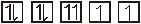(A) Hunds rule     (B) Aufbau rules        (C) n + l rules      (D) All of the above

Q.37    Difference between Cl a atom and Cl– ion of -
(A) Proton     (B) Electron         (C) Neutron     (D) Proton and electron

Q.38    Which element is represented by the following electronic configuration ?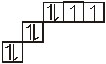(A) Nitrogen      (B) Oxygen         (C) Flourine     (D) Neon

Q.39    Which of the following electronic configuration does not obey aufbau principal.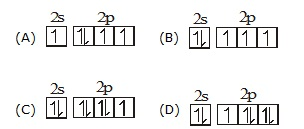Q.40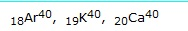(A) Isotopes     (B) Isobars         (C) A & B both     (D) None

Q.41    Electronic configuration of Fe+2 is -
(A) (Ar) 4s2 3d6     (B) (Ar) 3d6         (C) (Ar) 4s23d4    (D) (Ar) 4s23d0

Q.42    The charge and mass of the proton present in the nucleus of helium atom are respectively-
(A) +2, 4u    (B) +1, 1u         (C) +2, 1u    (D) +2, 2u

Q.43    Which of the following is not correct observation/conclusion from Rutherford scattering experiment-
(A) Nucleus is small but heavy
(B) Nucleus always carries positive charge
(C) Atom is nearly 105 times greater  than the size of the nucleus
(D) The number of a-particle hiting the nucleus is very large.

Q.44    Neutron is present in all atom except-
(A) Protium     (B) Deuterium         (C) Tritium    (D) Helium

Q.45    A monovalent anions has 10 electrons and 10 neutrons. The atomic number and mass number of the element are respectively-
(A) 10, 20              (B) 9, 18        (C) 10, 20        (D) 9, 19

Q.46    A trivalent cation of an element contains 10 electrons. The atomic number of the element is-
(A) 10               (B) 7             (C) 13              (D) None of these

Q.47    Which of the following isotope is used in the treatment of blood cancer -
(A) P-32    (B) I-131         (C) Co-60    (D) Any one of these

Q.48    Which isotope is generally used as a nuclear fuel-

(A) U-235    (B) Co-60        (C) P-32     (D) Iodine

Q.49    Which isotope is used in treatment of cancer ?
(A) Co-60          (B) U-235        (C) P-32      (D) Iodine

1.     B    2.     B     3.       B    4.   A
5.     C     6.     B     7.       B    8.    B
9.     A    10.   A    11.   C    12.  D
13.   B    14.   C    15.     A   16.   C
17.   B    18.   B    19.     B   20.   D
21.  A    22.   D   23.     D  24.   C
25.   C    26.    C    27.   C   28.   A
29.   D    30.  C    31.     C    32.   D
33.   B    34.   B    35.     D   36.   D
37.   B    38.  B    39.     A   40.   B
41.    C    42  A    43.     D   44.   A
45.    D    46.  C   47.     A    48.   A
49.   A

## EXERCISE - II

### FILL IN THE BLANKS:

Q.1   Electron are ....................charged particle.
Q.2   The scientist who discovered the nucleus is ...................
Q.3   Neutron have mass equal to .....................but have .................electric charge.
Q.4   contain...........protons and ............neutrons.
Q.5  The atomic number is equal to the number of ................protons.
Q.6   An element has mass number 23, its atomic number is 11, the number of neutrons will be equal to ........
Q.7   Isotopes have same .............
Q.8   Isobars have same number of .............
Q.9  The charge on the atom containing 17 protons, 18 neutrons and 18 electrons is .............
Q.10  Neutrons are not present in ..................?
Q.11  17Cl35 and 17Cl37 are ......................?
Q.12  The maximum number of electrons that can be presented in the outermost shell an atom is.
Q.13  The total number of protons and neutrons in the nucleus of an atom is called its ...............
Q.14  The fundamental particles of an atom are...................and................
Q.15  Protons were discovered by ...............and electron................and neutrons by................
Q.16  What is the valency of fluorine........................
Q.17  Alpha-particle scattering experiment was responsible for the discovery of................

### TRUE OR FALSE

Q.1  Element having electronic arrangement
2, 8, 8,1,9 is Cu.
Q.2  Element having electronic arrangement
2, 8, 8, 1, 5 in chromium.
Q.3   In Na+ number of e– are more than the number of protons.
Q.4  Isotopes of iodine is used for making tincture of iodine in medicine.
Q.5  Neutron is sum of proton plus electron and therefore, it is neutral.
Q.6  Isobar of an element different in the number of neutron.
Q.7  Neutron were discovered by Hency Becqueral.
Q.8  Helium does not neutron.
Q.9   Hydrogen does not have neutron.
Q.10 The valency of potassium is 2.
Q.11 J.J. Thomson proposed that the nucleus of an atom contain only nucleons.
Q.12 A neutron is formed by an electron and a proton combining together. There, it is neutral.
Q.13 The mass of an electron is abouttimes that of proton.
Q.14 An isotoope of iodine is used for making tincture iodine, which is used as a medicine.

### FILL IN THE BLANKS

1.     –ve                      2.     Rutherford
3.     Proton, No          4.     6, 6
5.     Proton
7.     Atomic number
8.     Atomic mass        9.     –ve
10.     Hydrogen          11.     Isotopes
12.     8 Electron        13.     Atomic mass
14.     e,p and n
15.     Goldstein , JJ Thomson, Chadwick
16.     7                     17.     Nucleus

### True & False

1.     F    2.     T    3.     F    4.     T
5.     T    6.    T    7.     F    8.      F
9.     T    10.     F    11.     F    12.    T
13.    T    14.     T

### EXERCISE - III

Q.1    Metals and hydrogen are always :-
(A) Electropositive
(B) Electronegative
(C) Both A and B
(D) None of the above

Q.2    Mg+2 and F– ions differ in which of the following fundamental particles ?
(A) Electrons, protons and neutrons        (B) Protons and neutrons
(C) Only protons                                      (D) Neutrons and electrons

Q.3    Valency of iron in FeCl2 and FeCl3 is _______ and _______ respectively
(A) 1, 2        (B) 2, 1            (C) 2, 3        (D) 3, 2

Q.4    Valency of tin in SnCl2 and SnCl4 is _______ and _______ respectively

(A) 2, 4        (B) 4, 2            (C) 3, 4        (D) 2, 3

Q.5    Valency of tin in SO2 and SO3 is _______ and _______ respectively
(A) 3, 6        (B) 2, 3            (C) 2, 6        (D) 4, 6

Q.6    The charge carried by an electron is :-
(A) 1.602 × 1019 coluombs            (B) 1.602 × 10–19 coluombs
(C) 1.609 coluombs                       (D) 6.02 × 1019 coluombs

Q.7    Which of the following electronic configuration is wrong ?
(A) Be (3) = 2, 1
(B) O (8) = 2, 6
(C) S (16) = 2, 6, 8
(D) P (15) = 2, 8, 5

Q.8    The mass of a proton is :-
(A) 1.609 g        (B) 1.6 × 1024 g    (C) 1.6 × 10–23 g    (D) 1.6 × 10–24 g

Q.9    Which of the following electronic configuration represents a noble gas ?
(A) 2, 8, 2        (B) 2, 8, 6        (C) 2, 8        (D) 2, 8, 8, 2

Q.10    The e/m is not constant for :-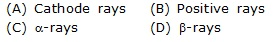Q.11    Size of the nucleus is :-
(A) 10–15 cm        (B) 10–13 cm        (C) 10–10 cm        (D) 10–8 cm

Q.12    Nucleus model of the atom was proposed
by :-
(A) Thomson        (B) Neils Bohr        (C) Moseley        (D) Rutherford

Q.13    Cathode rays have :-
(A) Only mass                                   (B) Only charge
(C) Neither mass nor charge            (D) Both mass and charge

Q.14    Which of the following is false ?
(A) Neutron has highest mass among fundamental particles
(B) The mass of an electron is negligible
(C) e/m is highest for a proton
(D) Charge of neutron is zero

Q.15    The ratio of specific charge of a proton and a particle is :-
(A) 2 : 1        (B) 1 : 2        (C) 1 : 4        (D) 1 : 1

Q.16    Which of the following particles is the heaviest?
(A) Meson        (B) Electron        (C) Proton        (D) Neutron

Q.17    A species 'X' contains 9 protons, 10 electrons and 11 neutrons. It is :-
(A) A neutral atom    (B) An isotope        (C) A cation        (D) An anion

Q.18    Bohr's atomic theory gave the idea of :-
(A) Quantum numbers                (B) Shape of sublevels
(C) Nucleus                    (D) Stationary states

Q.19    Energy of an orbit :-
(A) Increases as we move away from the nucleus
(B) Remains same as we move away from the nucleus
(C) Decreases as we move away from the nucleus
(D) None of the above

Q.20    According to Bohr's model of hydrogen atom :-
(A) The linear velocity of the electron is quantised
(B) The angular velocity is quantised
(C) The linear momentum of the electrons is quantised
(D) The angular momentum of the electron is quantised

Q.21    If three neutrons are added to the nuclei of 92U235, the new nucleus will have an atomic number of :
(A) 89            (B) 95            (C) 90            (D) 92

Q.22    Oxygen atom and oxide ion have :-
(A) Same size                                            (B) Same electron number
(C) Same electronic configuration              (D) Same proton number

Q.23    Which of the following statement is true ?
(A) F– has more electrons than Na+        (B) F– has less electrons than Na+
(C) Na+ and F– has equal electrons         (D) None of the above

Q.24    Which of the following ions is the smallest in size ?
(A) Mg2+        (B) Na+            (C) O2–        (D) F–

Q.25    The maximum number of electrons that can be accommodated in the 4th energy level is :-
(A) 32            (B) 18            (C) 2            (D) 8

Q.26    Size of an atoms is expressed in terms of :-
(C) Ionic radius                    (D) Either A or B

Q.27    The metals of s-block elements combine directly with halogens (X) at appropriate temperature to form halides of the :-
(A) MX and MX2 type                (B) MX2 and MX3 type
(C) MX3 and MX4 type             (D) MX and MX3 type

Q.28    The total number of electrons in a nitrogen atom and chlorine atom is 7 and 17 respectively. Find the number of valence electrons in them :-
(A) 3, 1        (B) 5, 8            (C) 4, 1        (D) 5, 7

Q.29    Name the element having two electrons in the K shell of its atom :-

(A) Hydrogen        (B) He+            (C) Helium        (D) None of these

Q.30    Isotopes differ in :-
(A) Physical properties                (B) Chemical properties
(C) Number of protons                (D) None of these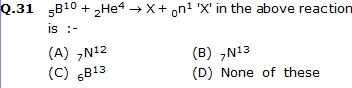Q.32    On the bombardment of 7N14 with a-particles, the nucleus of the product after the release of a proton will be :-
(A) 8O17        (B) 8O18        (C) 9F17        (D) 9F18

Q.33    The isotope used for dating archaeological findings is :-
(A) 1H3        (B) 6C14        (C) 8O18        (D) 92U235

1.     A    2.     B     3.     C    4.     A
5.     D    6.     B    7.     C    8.      D
9.     C    10.    B    11.    A    12.    D
13.    D    14.   A    15.    B    16.    C
17.    D    18.   A    19.    A    20.    D
21.    D    22.   D    23.    C    24.   A
25.    A    26.   A    27.    A    28.   D
29.    C     30.   A   31.     B    32.    A
33.    B

Q.1    A proton is identical to-
(A) the nucleus of helium
(B) the nucleus of a hydrogen atom
(C) a molecule of a hydrogen
(D) an atom of hydrogen

Q.2    An a-particle is-
(A) a hydrogen molecule
(B) a helium nucleus
(C) an electron
(D) a proton

Q.3    The atomic number of an element is determined by-
(A) the number of electrons in one atom    (B) the number of neutrons in one atom
(C) the valency of the element
(D) the number of protons in one atom

Q.4    The value of e/m of an electron was measured by-
(A) Millikan
(B) J.J. Thomson
(C) Dalton
(D) Rutherford

Q.5    The atomic number of an element is 11 and its mass number is 23. The respective number of electrons, protons and neutrons in this atom will be:
(A) 11, 11, 12
(B) 11, 12, 11
(C) 12, 11, 11
(D) 23, 11, 23

Q.6    The number of electrons in the outer shell of the most stable or inert atoms is:
(A) 1         (B) 4              (C) 6                 (D) 8

Q.7    Which of the following pairs are isotopes?
(A) oxygen and ozone
(B) ice and steam
(C) nitric oxide and nitrogen dioxide    (D) hydrogen and deuterium

Q.8    An atom which has a mass number of 14 and has 8 neutrons is an:
(A) isotope of oxygen
(B) isobar of oxygen
(C) isotope of carbon
(D) isobar of carbon

Q.9    Which of the following has an equal number of neutrons and protons?
(A) hydrogen
(B) deuterium
(C) fluorine
(D) chlorine

Q.10    An atom of an element has 26 electrons atom contains _________ neutrons.
(A) 26    (B) 36
(C) 30    (D) 56

Q.11    For an element with atomic number 19, the 19th electron will occupy-
(A) L-shell
(B) M-shell
(C) N-shell
(D) K-shell

Q.12    Members of which of the following have similar chemical properties?
(A) isotope
(B) isobars
(C) allotropes
(D) both isotopes and allotropes

Q.13    The number of of electrons in an element with atomic number X and mass number Y will be-
(A) X – Y                 (B) Y – X                      (C) X + Y               (D) X

Q.14    A natural phenomenon that supports the experimental conclusion that atoms are divisible is-
(A) allotropy
(C) cracking
(D) none of these

Q.15    The relative atomic masses of many elements are not whole numbers because-
(A) they cannot be determined very accurately            (B) the atoms ionize during the determinations
(C) of the existence of isotopes                                 (D) of the presence of impurities

Q.16    The valency of an element is-
(A) the mass of the element displacing 1 part by the mass of hydrogen
(B) the mass of the element combining with 8 parts by mass of oxygen
(C) the number of atoms of hydrogen combining with 1 atom of the given element
(D) the number of atoms in 1 molecule of the given element

Q.17    The absolute charge of the electron is.
(A) 1.1 × 1011C
(B) 1.6 × 1014C
(C) 1.6 × 10–19
(D) 6.0 × 1021C

Q.18    The fundamental particles not present in the nucleus of hydrogen atom is-
(A) electron     (B) proton            (C) neutron      (D) none of these

Q.19    The number of valence electrons present in Ca atom-
(A) 3               (B) 2                     (C) 8                (D) 6

Q.20    The maximum number of electrons that can be accommodated in M Shell of an atom are-
(A) 8               (B) 32                  (C) 18               (D) 25

1.     D    2.     B    3.     D    4.     A
5.     A    6.     D    7.     D    8.     C
9.     B    10.    C    11.     B    12.   A
13.    D    14.    B    15.    C    16.   C
17.    C    18.    C    19.     B    20.  C

### IIT-JEE QUESTIONS

1.    The 'nuclear model' of atom was proposed by-
(A) Aston     (B) Bohr    (C) Chadwick    (D) Rutherford

2.    Which of the following species has no charege -
(A) Electron     (B) Neutron     (C) Nucleus     (D) Proton

3.    The nucleus of an atom contains -
(A) Neutrons and electron     (B) Protons and electrons
(C) Protons and neutrons   ,   (D) Protons, neutrons and electrons

4.    Neutrons were discovered by -
(A) Aston    (B) Bohr    (C) Chadwick     (D) Rutherford

5.    According to the nuclear model of atom -
(A) Electron and neutrons are present in the centre and protons are present in the outer space
(B) Electron and protons are present in the centre and neutrons are present in the outer space
(C) Protons and neutrons are present in the centre and electrons are present in the outer space
(D) Neutrons are present in the centre while protons and electrons are present in the outer space.

6.    The element with the same atomic number and mass number is-
(A) Oxygen     (B) Hydrogen     (C) Helium     (D) Carbon

7.    The mass of an electron is -
(A) 1837 times of mass of proton                     (B) 1/1837th times the mass of oxygen atom
(C) 1/1837th times the mass of carbon atom     (D) 1/1837th times the mass of hydrogen atom

8.    Neutrons were discovered by -
(A) Joseph Thomson     (B) James Chadwick    (C) Ernest Rutherford    (D) John Dalton

9.    The scientist who put forward the atomic theory in 1808 was
(A) Dalton     (B) Lavoiser    (C) Galileo    (D) Thomson

10.    The mass number of an element is the sum of the number of -
(A) Proton and electron                            (B) Electron and neutrons
(C) Protons, electrons and neutrons         (D) Protons and neutrons

11.    Rutherford directed a stream of .........on a thin Gold leaf in the scattering experiment-
(A) Alpha particle          (B) Beta particle
(C) Gamma particle         (D) X-rays

12.    Atom is electrically neutral because -
(A) It contains neutrons
(B) It contains euqal number of protons and electrons
(C) The electrons are in a state of continuous motion around the nucleus
(D) The nuclues in the centre of the atom

13.    The mass of an electron is-
(A) Equal to that of a neutron    (B) Equal to that of proton
(C) Greater that of a proton      (D) 1/1840 that of a proton

14.    The mass of an electron is -
(A) 9.11 × 10–29kg    (B) 9.11 × 10–31kg    (C) 9.11 × 10–33kg    (D) 9.11 × 10–35kg

15.    A unit of charge is equal to -
(A) 1.602 × 10–19C    (B) 1.602 × 10–20C    (C) 1.602 × 10–21C    (D) 1.602 × 10–22C

16.    The sizes of a cation and the corresponding atom bear the following relationship-
(A) rcation > ratom
(B) rcation < ratom
(C) rcation = ratom
(D) rcation may be greater than or smaller than or equal to raction depending upon the nature of cation and     atom

17.    The sizes of an anion and the corresponding atom bear the following relationship-
(A) ranion > ratom
(B) ranion < ratom
(C) ranion = ratom
(D) ranion may be greater than or smaller than or equal to ratom depending upon the nature of cation and         atom

18.    In the nth electronic level, the number of orbitals is -
(A) n              (B) 2n               (C) n2       (D) 2n2+1

19.    Which of the following orbitals is not possible -
(A) 1s             (B) 1p               (C) 2p        (D) 3d

20.    Which of the following orbitals has larger energy -
(A) 2s             (B) 2p              (C) 1s     (D) 3p

21.    Which of the following orbitals has spherical shape-
(A) s               (B) p              (C) d       (D) f

22.    Atomic number of an element is denoted by -
(A) X            (B) A           (C) Z          (D) N

23.    In the periodic table, the elements are arranged in the -
(A) Increasing order of atomic mass         (B) Decreasing order of atomic number
(C) Alphabetical order                              (D) Increasing order of atomic number

24.    In a neutral atom, the number of proton is equal to -
(A) Number of electron     (B) Half the number of electrons
(C) Number of neutrons     (D) Number of electrons + number of protons

25.    The mass number of an element is denoted by
(A) A                 (B) Z            (C) X          (D) N

26.    The mass proton is about the same as that of -
(A) Carbon atom     (B) An electron    (C) Hydrogen ion     (D) Oxygen atom

27.    Mass of a neutron is equal to -
(A) The mass of an electron     (B) Half of mass of an electron
(C) The mass of proton     (D) Half of mass of a proton

28.    The isotope possesss
(A) Similar physical properties    (B) Similar chemical properties
(C) Different physical properties     (D) Different chemical properties

29.    Cathode rays are
(A) Alpha particles    (B) Electrons     (C) Neutrons     (D) Protons

30.    From the discharge tube experiment in which cathode rays are emitted, it is inferred that
(A) Mass of a proton is in fraction     (B) matter contains electrons
(C) Nucleus is postively charged         (D) Positive rays are heavier than protons

31.    The flurescence on the wall of discharge tube is due to-
(A) Alpha rays     (B) anode rays     (C) Canal rays    (D) Cathode rays

32.    Which of the following shells can have a maximum number of 18 electrons
(A) K-shell            (B) L-shell        (C) M-shell        (D) N-shell

33.    Mass number of an element is equal to the
(A) Number of neutrons
(B) Number of protons
(C) Sum of the numbers of protons and neutrons in the nucleus
(D) Sum of the numbers of protons and electroons

34.    Which of the following is not true for isotopes -
(A) They have the same atomic number    (B) They have the same mass number
(C) They have the same electornic configuration
(D) The have the same chemical properties

35.    In ,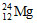the number 24 represents -
(A) Atomic number    (B) Mass number    (C)  Number of neutrons     (D) Number of electrons

36.    Which of the following properties of atoms of elements is definitely a whole number -
(A) Relative atomic mass      (B) Atomic volume
(C) Atomic number              (D) Atomic radius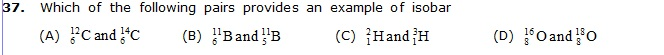38.    In the nth electronic  level, the number of electrons is given by -
(A) n                  (B) 2n      (C) 2n2     (D) 2n2 + 1

39.    The mass number of helium nucleus is -
(A) 1             (B) 2         (C) 4    (D) 6

40.    The number of electrons in 13Al3+ is -
(A) 13            (B) 12      (C) 11     (D) 10

41.    The approximate radii of the nuclei of atoms lie in the range of
(A) 10–14 – 10–15m    (B) 10–12 – 10–11m    (C) 10–11 – 10–10m     (D) 10–28 – 10–29m

42.    The approximate radii of atoms lie in the range of -
(A) 10–6m – 10–5m    (B) 10–7m – 10–6m     (C) 10–18m – 10–17m    (D) 10–10m – 10–9m

43.    Most favourable for electrovalency are-
(A) Low charge on ions, large cation and small anion
(B) High charge on ions, small cation and large anion
(C) High charge on ions, large cation and small anion
(D) Low charge on ions, small cation and large anion

44.    When sodium chloride is dissolved in water, the sodium ion gets -
(A) Oxidised     (B) Reduced      (C) Hydrolysed     (D) Hydrated

45.    Which one of the following bonds will be the most polar-
(A) N–Cl            (B) O – F             (C) C – F          (D) N – N

46.    Rutherford a-particle scattering experiement eventually led to the conclusion that
(A) mass and energy are related                            (B) electrons occupy space around the nucleus
(C) neutrons are burried deep in the nucleus         (D) the point of impact with matter can be precisely determined.

47.    Atomic number of an element is equal to the -
(A) Number of electrons in the atom    (B) Number of nucleons in the atom
(C) Number of neutrons in the atom     (D) Total number of elementary particle in the atom

48.    Sodium atom differs from sodium ion in the number of -
(A) Electrons    (B) Nucleons      (C) Neutrons      (D) Protons

49.    Calcium atom and calcium ion differ in all of the following respects except-
(A) Net electrical charge      (B) Number of electrons
(C) Number of protons        (D) Radius

50.    Neutrons is not present in -
(A) Helium     (B) Deuterium     (C) Protium     (D) Tritium

51.    Number of electron outside the nucleus of an atom is equal to the number of
(A) Neutrons in the nucleus     (B) Protons in the nucleus
(C) Neutrons minus protons in the nucleus (D) Neutrons plus protons in the nucleus

52.    Isotopes differ in their
(A) Chemical reactivity     (B) Number of neutrons
(C) Number of protons     (D) Valence electrons

53.    The number of electrons in the atom which has 17 protons in the nucleus is -
(A) 10          (B) 17      (C) 34      (D) Not predictable

54.    Which of the following is not true of isobars
(A) They have different mass number    (B) They have different atomic number
(C) They have different number of protons
(D) They have different electronic configurations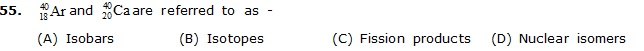(A) Isobars    (B) Isotopes     (C) Fission products     (D) Nuclear isomers

56.    Which of the following has more electrons than neutrons -
(A) Na+    (B) Mg2+    (C) F–    (D) O2–

57.    In , the number 3 represents
(A) Atomic number    (B) Relative atomic mass (C) Mass number    (D) Number of neutrons

58.    Which of the following sub-atomic particles are always present in the nuclei of atoms
(A) Electrons     (B) Alpha particles    (C) Positrons      (D) Protons

59.    Mass number of is-
(A) 17    (B) 35     (C) 35–17=18     (D) 35 + 17 = 52

60.    Atomic number of is-
(A) 11     (B) 23    (C) 23 – 11 = 12    (D) 23 + 11 = 34
Instruction :
The question from 61 to 63 consist of an Assertion (a) and Reason (r). Use the following key to choose the correct answer-
(A) If both (a) and (r) are correct and (r) is the correct explanation of (a)
(B) If both (a) and (r) are correct and (r) is not the correct explanation of (a)
(C) If (a) is correct but (r) is incorrect
(D) If (a) is incorrect but (r) is correct

61.   (a) : In Rutherford gold foil experiment, very few a-particles are deflected black
(r) : Nucleus present inside the atom is heavy

62.   (a) : Ionic compounds tend to be non-volatile.
(b) : Inter molecular forces in these compounds are weak

63.    (a) The atom in a covalent molecule are said to share electrons, yet some are polar.
(r) : In polar covalent molecule, the shared electron spend more time on the average near one of the atoms.

64.    Two atoms A and B are represented by their mass number and atomic number as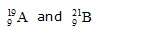(A) A and B belong to the same element     (B) A and B have the same number of protons
(C) A and B are isotopes                             (D) A and B are isobars
(E) A and B have the same number of neutrons
Which one of the following groups of statement in correct-
(A) c, e, d    (B) a, b, d     (C) b, c, a    (D) e, a, b

65.    Mass number of oxygen is -
(A) 8             (B) 16      (C) 20             (D) 32

66.    Which of the following species are isoelectronics-
(A) He and Ne         (B) Cl– and Br–      (C) Na+ and Mg2+    (D) Mg2+ and Ca2+

67.    has
(A) 17 protons and 17 neutrons     (B) 17 protons and 20 neutrons
(C) 17 protons and 37 neutrons    (D) 37 protons and 17 neutrons

68.    The addition of a nuetrons to the nucleus of an atoms result in -
(A) Increase in atomic number    (B) Increase in mass number
(C) Increase in nuclear charge     (D) Formation of an isobar

69.    Na is the symbol of -
(A) Sulphur    (B) Sodium      (C) Potassium      (D) None of these

70.    The atomic and mass number of Helium are-
(A) 6, 12            (B) 7, 14      (C) 2, 4      (D) 15, 31

71.    The symbol of phorphorus is -
(A) K             (B) P         (C) F       (D) Fe

72.    Atoms of same element having same atomic number but different mass number is called-
(A) Isotope     (B) Isobar    (C) Isoelectronic     (D) None of these

73.    Atomic number 6 and mass number 12 are of the element
(A) Hydrogen     (B) Nitrogen     (C) Carbon      (D) Oxygen

74.    are the ...........of hydrogen
(A) Isotope    (B) Isobar     (C) Both isotope and isobar (D) Allotrops

75.    Carbon has three isotopes, whose mass number are -
(A) 11, 12, 13    (B) 12, 13, 14     (C) 14, 15, 16     (D) 6, 7, 8

76.    Atom of different element with different atomic number but same mass number are called.
(A) Allotropes    (B) Isobar    (C) Isotope     (D) None of these

77.    Protium, deuterium and tritium are the isotopes of -
(A) Nitrogen     (B) Oxygen     (C) Hydrogen     (D) Carbon

78.    Entire positive charge in an atom is concentrated in a small area at its centre called nucleus. This was suggested by -
(A) Thomson    (B) Rutherford    (C) Bohr     (D) Dalton

79.    Which is the correct order of orbital energy in a multi-electron atom
(A) 1s > 2s < 2p < 3s < 3p < 3d     (B) 1s < 2s < 2p < 3s < 3p < 3d
(C) 1s > 2s < 3d < 2p < 3s < 3p     (D) 1s > 2s < 2p < 3s < 3p < 3d

80.    Which of the following configuration represents more electropositive atom ?